# Challenge Exercises on Sets

 2. Which of the following statements is true about the set listed below? C = {prime numbers less than 20} 9C -2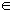C C is an infinite set. All of the above. RESULTS BOX:
 3. Which of the following is a finite set?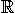{ x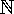: x  ≥  2 and x ≤ 6  } A = {0, 1, 2, 3, ...} B = {-3, -2, -1, 0, 1, 2, 3, 4, 5, ...} RESULTS BOX:
 4. Which of the following sets is equal to the set given below?  { n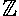| -3  ≤  n  < 7 } D = {..., -3, -2, -1, 0, 1, 2, 3, 4, 5, 6, ...} E = {-2, -1, 0, 1, 2, 3, 4, 5, 6, 7} F = {-3, -2, -1, 0, 1, 2, 3, 4, 5, 6} All of the above. RESULTS BOX:
 6. If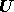= {whole numbers less than 20} and P = {prime numbers less than 20}, then which of the following sets overlaps with P,and is also a part of? Q = {even numbers less than 20} R = {multiples of 4} S = {factors of 36} All of the above. RESULTS BOX:
 7. Which of the following accurately explains the meaning of the set listed below? { y:  y ≤ -3 } The set of all y insuch that y is any number greater than or equal to -3. The set of all y insuch that y is any number less than or equal to -3. The set of all y insuch that y is any number less than -3. None of the above. RESULTS BOX:
 8. Which of the following statements is true?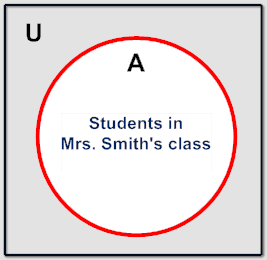A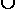A' =A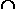A' = Ø A' = {Students not in Mrs. Smith's class} All of the above. RESULTS BOX:
 9. If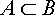, then which of the following statement is true? A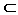BØA All of the above. RESULTS BOX:
 10. If, then which of the following statement is true? AB = B AB = B AB = C All of the above. RESULTS BOX: